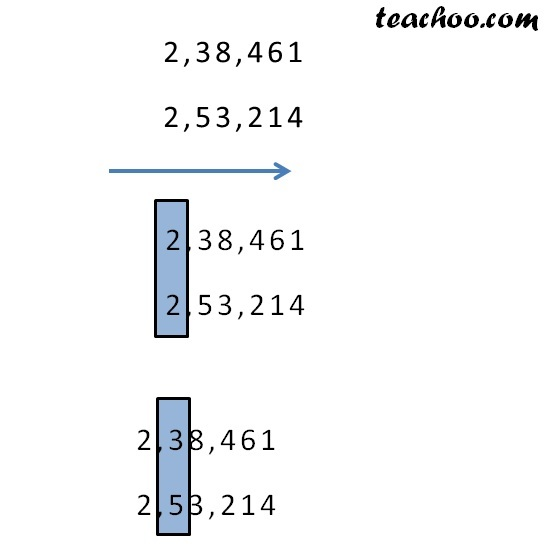Comparing large numbers

Chapter 1 Class 6 Knowing our Numbers
Concept wise

Which number is larger?

238461  &  53214

First, put numbers one below the other

#### 53,214

Since 2,38, 461 has more digits are than 53, 214

∴ 2,38, 461 is larger than 53, 214

Which number is larger?

238461  &  253214

First, put numbers one below the other

2,38,461

2,53,214

Since number of digits are same,

we compare individual digits

starting from left to rightSince

2 = 2

So, we compare the next digit

3 < 5

So,    2,38,461 < 2,53,214

∴ 2,53,214 is larger

Learn in your speed, with individual attention - Teachoo Maths 1-on-1 Class

### Transcript

Comparing Large Numbers Which number is larger? 238461 & 53214 First, put numbers one below the other 2,38,461 53,214 Since 2,38, 461 has more digits are than 53, 214 ∴ 2,38, 461 is larger than 53, 214 Which number is larger? 238461 & 253214 First, put numbers one below the other 2,38,461 2,53,214 Since number of digits are same, we compare individual digits starting from left to right 2,38,461 2,53,214 2,38,461 2,53,214 2 = 2 So, we compare the next digit 2,38,461 2,53,214 3 < 5 So, 2,38,461 < 2,53,214 ∴ 2,53,214 is larger